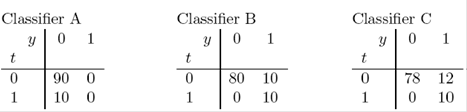# How to measure a classifier”s quality. You”ve just written a new classification algorithm and wan

How to measure a classifier”s quality. You”ve just written a new classification algorithm and want to measure how well it performs on a test set, and compare it with other classifiers. What performance measure should you use? There are several standard answers. Let”s assume the classifier gives an output y(x), where x is the input, which we won”t discuss further, and that

the true target value is t. In the simplest discussions of classifiers, both y and t are binary variables, but you might care to consider cases where y and t aremore general objects also.

The most widely used measure of performance on a test set is the error rate { the fraction of misclassifications made by the classifier. This measure forces the classifier to give a 0/1 output and ignores any additional information that the classifier might be able to offer { for example, an indication of the  firmness of a prediction. Unfortunately, the error rate does not necessarily

measure how informative a classifier”s output is. Consider frequency tables showing the joint frequency of the 0/1 output of a classifier (horizontal axis),and the true 0/1 variable (vertical axis). The numbers that we”ll show are percentages. The error rate e is the sum of the two o_-diagonal numbers, which we could call the false positive rate e+ and the false negative rate e??.

Of the following three classifiers, A and B have the same error rate of 10%and C has a greater error rate of 12%.But clearly classifier A, which simply guesses that the outcome is 0 for all cases, is conveying no information at all about t; whereas classifier B has an informative output: if y = 0 then we are sure that t really is zero; and if y=1then there is a 50% chance that t=1, as compared to the prior probability(t=1) = 0,1. Classifier C is slightly less informative than B, but it is still much more useful than the information-free classifier A. One way to improve on the error rate as a performance measure is to report the pair (e+, e), the false positive error rate and the false negative error rate, which are (0;,0,1) and (0,1, 0) for classifiers A and B. It is especially important to distinguish between these two error probabilities in applications where the

two sorts of error have different associated costs. However, there are a couple of problems with the `error rate pair”: First, if I simply told you that classifier A has error rates (0, 0,1) and B

has error rates (0,1, 0), it would not be immediately evident that classifier is actually utterly worthless. Surely we should have a performance measure that gives the worst possible score to A!